Adding Calculated Quiz Questions Article Last Updated June 2021 For questions involving numeric calculations, the calculated question types on eClass can help to ensure that each student sees a different version of each question. For example, you could have a question that asks “what is the sum of X and Y” where X and Y are randomly generated for each student and the correct answer is automatically calculated and graded by eClass. There are 3 types of calculated questions that can be added to eClass quizzes: Calculated Questions require students to submit a numeric answer (optionally with units). Calculated Multichoice Questions are presented to students in a multiple choice format. Calculated Simple Questions are similar to Calculated questions but with a more narrow feature set and easier setup. Regular Calculated questions are described in detail below. Most of the setup for Calculated questions can be applied to the other two calculated questions types. The differences in setup are mentioned at the bottom of this article. Basic instructions on adding questions to quizzes can be found in Adding and Managing Quiz Questions.   Calculated Setting up calculated questions is a 3-step process. First you need to set up the question text and formula used to calculate the correct answer, then the properties of the dataset must be defined, and lastly you need to generate and test the random wild card values to be used in the question. Entering Question Text and Answers: The question text is what the student will see when they view the question. In places where there should be a randomized number, place a unique identifier inside of curly braces as a placeholder. These are called wild cards (e.g. “What is the sum of {A} and {B}?”).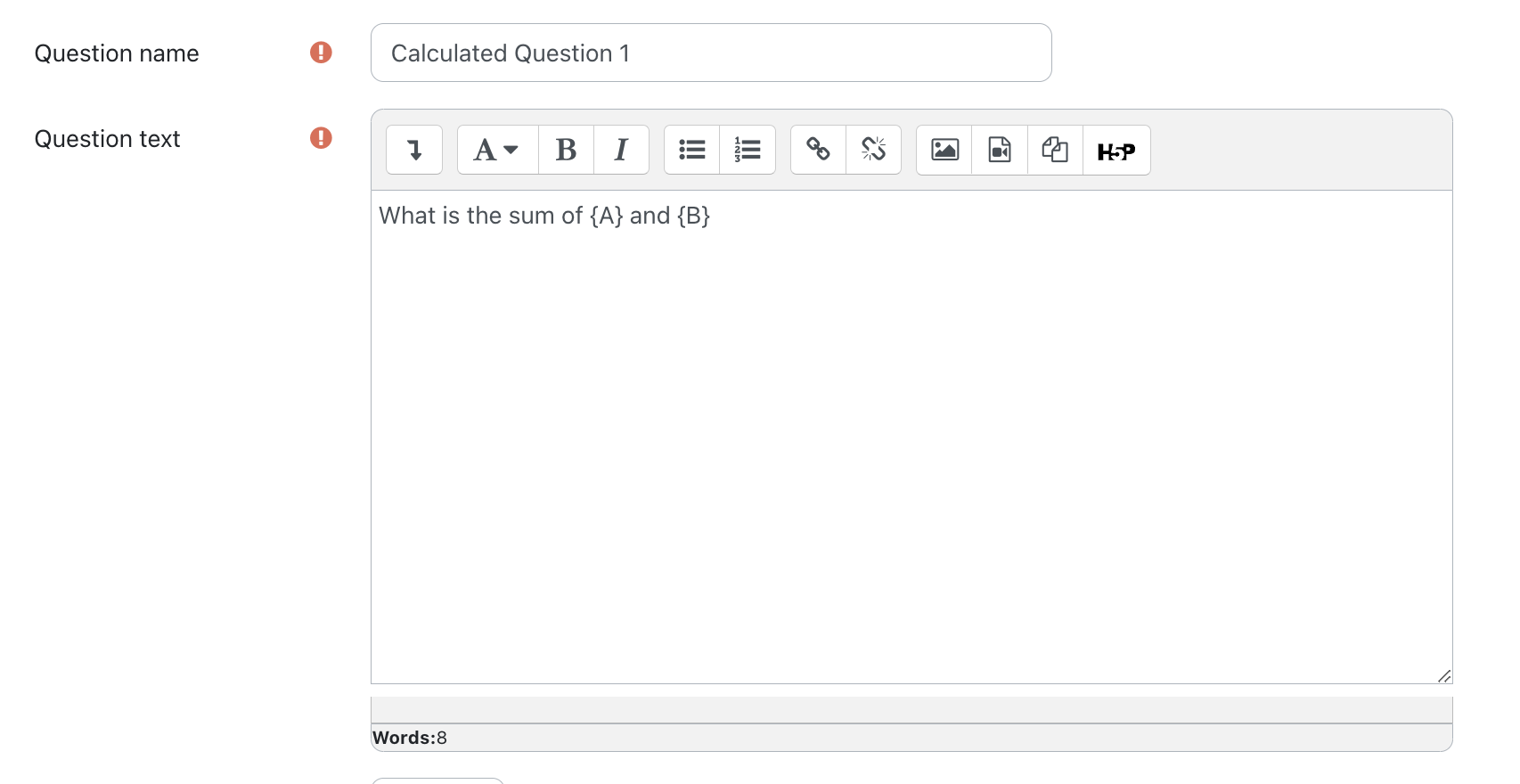Under Answers, the Answer formula is used to calculate the correct answer based on the wild cards in the question. For the above example, the answer formula would be “{A} + {B}”. Make sure to set the Grade for the correct answer to 100%. Additional answers can be added and their grades adjusted if you wish to give partial credit for different answers or tolerances.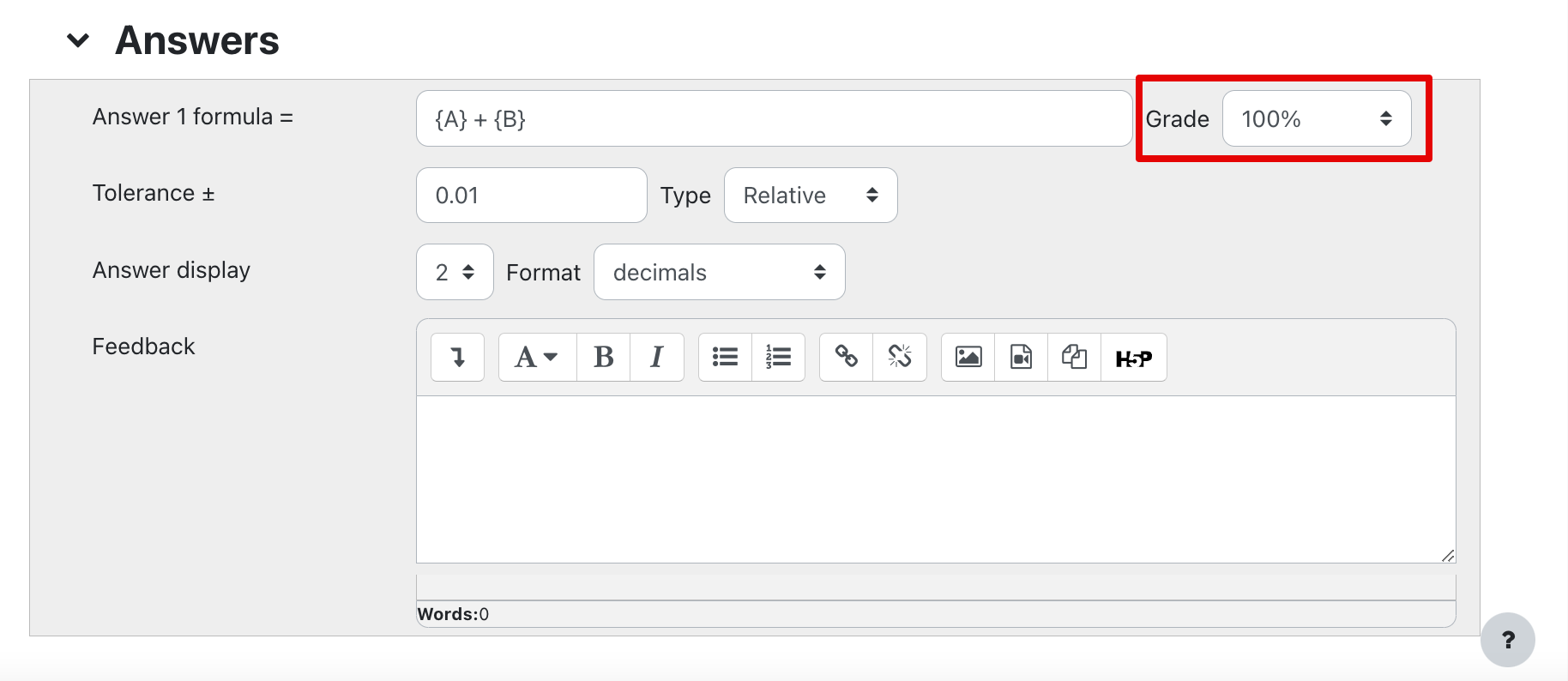There are three types of Tolerance that can be selected for an answer. The following example calculations are based on the assumption that the correct answer is 200 and the tolerance is set to 0.5: Relative: 200 ± 0.5 * 200 Nominal: 200 ± 0.5 Geometric: Upper limit: 200 + 0.5 * 200 Lower limit: 200 / (1 + 0.5) Nominal is generally the most straightforward method to use. Relative can be useful when different wild card values can result in a broad range of answers across multiple orders of magnitude. Geometric allows for a tolerance greater than 1 without the lower limit becoming less than 0. Saving changes will take you to the dataset properties. Setting the Dataset Properties: Datasets define what values will be used for each wild card. Shared datasets can be used when you are creating a number of similar questions. For example, if you are creating several questions on circle geometry, you could have a wild card for {radius} and use the same dataset for all of the questions that use the wild card {radius}. When writing/editing a question, existing shared datasets are displayed at the top of the page.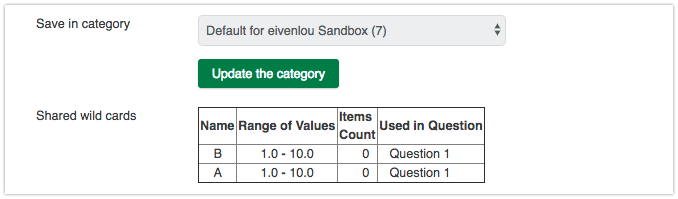Private datasets should be used when a dataset will only be used in one question.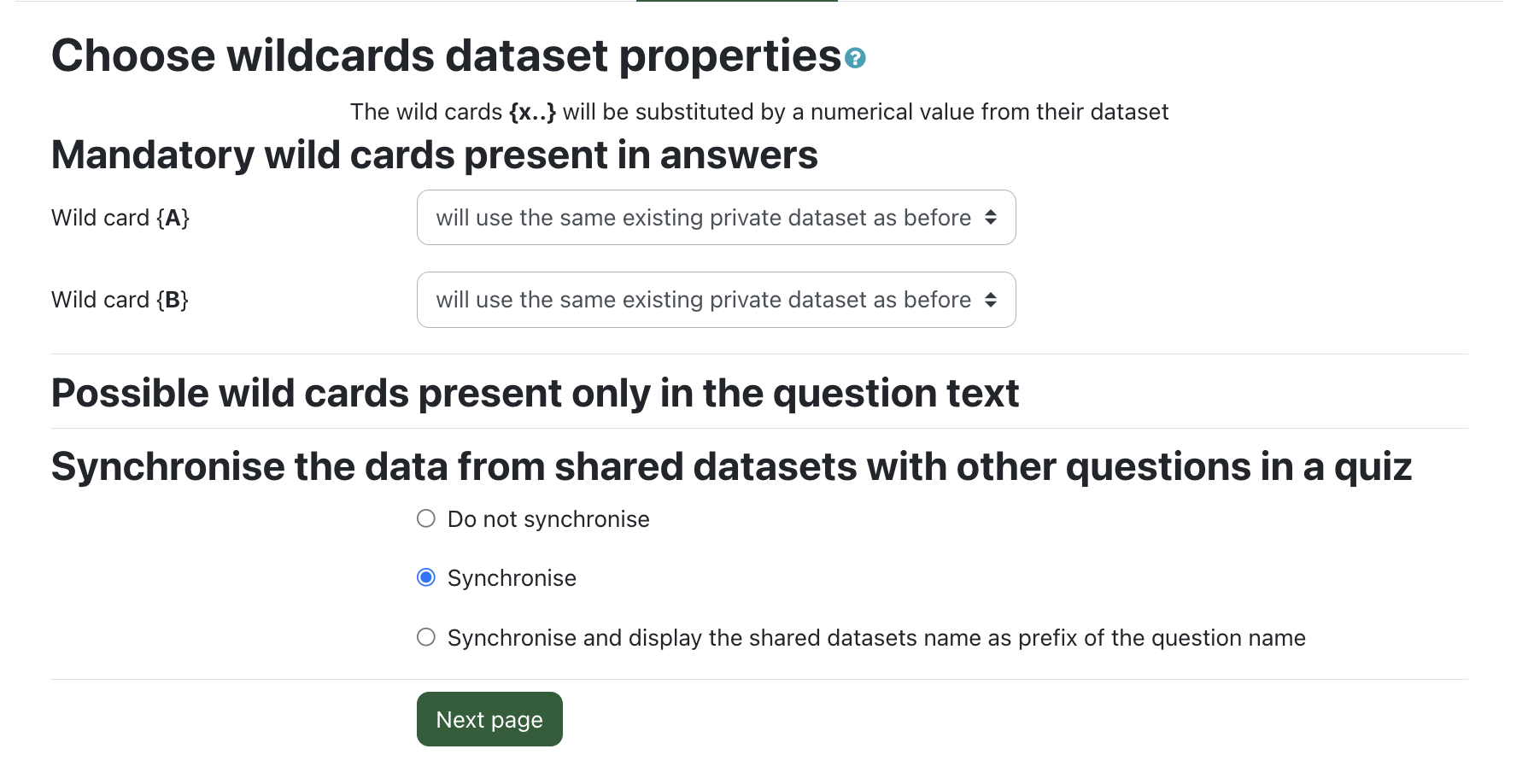If you have question text which appears to be a wild card (enclosed in curly braces) but is not used in the question’s answer, you will be asked to confirm whether or not it is a wild card. Synchronization allows you to use the same randomly generated numbers across multiple questions. For example, in question 1 you could define the dimensions of a cube and ask for the surface area. Then, by enabling synchronization for questions 1 and 2, you could have question 2 ask for the volume of the same cube. If synchronization is not enabled for a question then it will randomly generate wild cards independent from other questions in the quiz - even if they share a dataset. If you are not synchronizing or sharing datasets, using the Calculated simple question type (see below) may make it easier to setup questions. Otherwise, selecting Next page will allow you to edit your datasets. Editing Datasets: The random numbers used to replace wild cards must be generated and checked before being used in the question. Under Item to add you can select the parameters for the wild cards to be generated. Next, under Add, select the number of unique questions you would like generated from the dropdown and then press the Add button. If there are no errors, you can Save changes at the bottom of the page. If there is an error that the correct answer is outside the limits of true value, you need to update the answers tolerance parameters or increase the number of decimals/significant figures displayed in the answer. Both of these values can be modified underneath Answers tolerance parameters by selecting Show more. After making changes, the Update the answers tolerance parameters button can be used to save the new tolerance parameters and re-check the generated wild cards.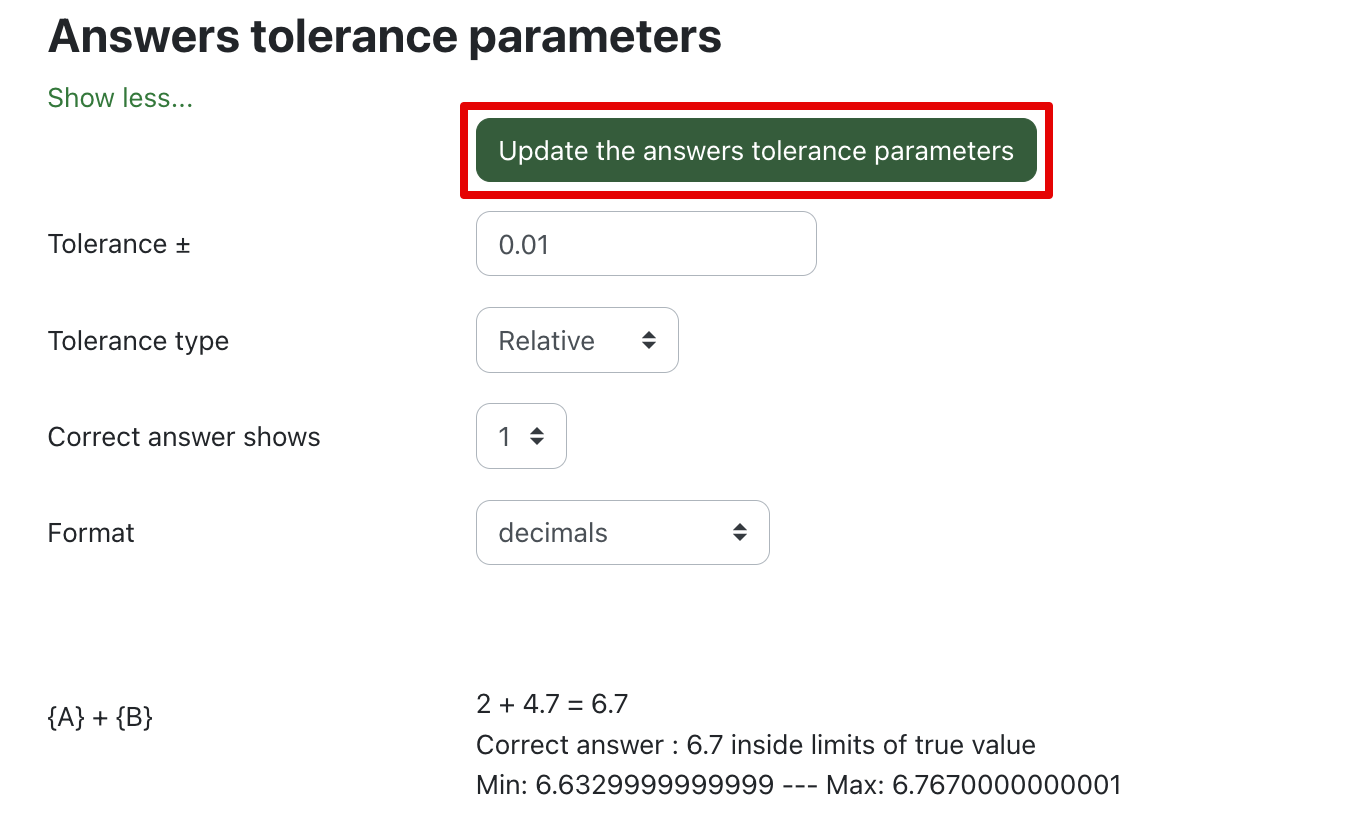Calculated multichoice Calculated multichoice questions have the same features and 3-step setup as regular calculated questions but are in a multiple choice format. Choices are written in the following format:     The perimeter is { = 2 * {width} + 2 * {height} } cm. Parts of a choice that should be calculated are preceded by an equals sign and enclosed in curly braces. Text can also be incorporated (outside of the curly braces) which can be useful for denoting units.   Calculated simple Simple calculated questions are the same as regular calculated questions except for they have a simpler interface for defining wild card datasets. For these questions, everything is setup on a single page (compared to regular calculated questions which have a 3-page setup). Simple calculated questions cannot take advantage of shared or synchronized wild card datasets. Generating wild cards: After writing your question and answer(s), select the Find the wild cards {x…} present in the correct answer formulas button. Setup the Wild cards parameters used to generate the values. Select the Generate button to create new wild card values (this will overwrite any previously generated wild card values). The Display button and numeric dropdown list can be used to view the current wild card values. If you receive an error that the answer is outside the limits of true value, the answer’s tolerance or number of decimals/significant figures likely needs to be adjusted.   Notes Defining unit conversions allows students to submit their answers using a different unit and still be marked as correct. For example, if your accepted answer was 500m and you defined km with a multiplier of 0.001 then 500, 500m, and 0.5km would all be accepted as correct answers if units are set to optional. If units are required, 500m and 0.5km would be marked as correct and part marks would be awarded for just entering 500 (note that no marks would be awarded for submitting 0.5).   Known Issue with Decimal Places: Datasets generated for Calculated Questions in Quizzes will continue to produce values with decimal places despite setting values to contain no decimals.(1 vote(s))HelpfulNot helpful